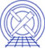AHELP for CIAO 3.4 bayes Context: sherpa

## Synopsis

A Bayesian maximum likelihood function.

## Description

In conventional fits involving background data, it is the best-fit background model amplitude in bin i, B(i), that is used when one attempts to ascertain the source model amplitude S(i). While this is the most probable value of B(i), it is not the only possible value, and S(i) may change if all possible values of B(i), weighted by their likelihood, are taken into account. This can be done using Bayes' Theorem. Below, we assume familiarity with the basics of Bayesian statistical methodology; a reader who is not familiar with these basics should consult, e.g., the review text by Loredo (1992, Statistical Challenges in Modern Astronomy, ed. Feigelson & Babu, 275).

For simplicity, assume that there are two fitting `regions' (in time, space, or both), denoted `off-source' and `on-source,' with N(i,B) and N(i,S) counts respectively. Counts are sampled from the Poisson distribution, and the best way to assess the quality of model fits is the use the Poisson likelihood function. Within the on-source region, the Poisson likelihood of the sum S(i) + B(i) is

`p[N(i,S) | S(i),B(i)] = [ [S(i)+B(i)]^N(i,S)/N(i,S)! ] * exp[-S(i)-B(i)] .`

We can relate this likelihood to the Bayesian posterior density for S(i) and B(i) using Bayes' Theorem:

`p[S(i),B(i) | N(i,S)] = p[S(i)|B(i)] * p[B(i)] * p[N(i,S) | S(i),B(i)] / p[D] .`

The factor p[S(i)|B(i)] is the Bayesian prior probability for the source model amplitude, which is assumed to be constant, and p[D] is an ignorable normalization constant. The prior probability p[B(i)] is treated differently; we can specify it using the posterior probability for B(i) off-source:

`p[B(i)] = [ A (A B(i))^N(i,B) / N(i,B)! ] * exp[-A B(i)] ,`

where A is an "area" factor that rescales the number of predicted background counts B(i) to the off-source region.

IMPORTANT: this formula is derived assuming that the background is constant asa function of spatial area, time, etc. If the background is not constant, the Bayes function should not be used.

To take into account all possible values of B(i), we integrate, or marginalize, the posterior density p[S(i),B(i) | N(i,S)] over all allowed values of B(i):

`p[S(i) | N(i,S)] = (integral)_0^(infinity) p[S(i),B(i) | N(i,S)] dB(i) .`

For the constant background case, this integral may be done analytically. We do not show the final result here; see Loredo. The function -log p[S(i)|N(i,S)] is minimized to find the best-fit value of S(i). The magnitude of this function depends upon the number of bins included in the fit and the values of the data themselves. Hence one cannot analytically assign a `goodness-of-fit' measure to a given value of this function. Such a measure can, in principle, be computed by performing Monte Carlo simulations. One would repeatedly sample new datasets from the best-fit model, and fit them, and note where the observed function minimum lies within the derived distribution of minima. (The ability to perform Monte Carlo simulations is a feature that will be included in a future version of Sherpa.)

### Note on Background Subtraction

The background should not be subtracted from the data when this function is used The background only needs to be specified, as in this example:

```sherpa> DATA 1 source.data
sherpa> BACK 1 background.data
sherpa> SOURCE 1 = [sourcemodel]
sherpa> STATISTIC BAYES
sherpa> FIT
...
```

To compare the best-fit source model with the data in a plotting environment, one would have to subtract the background from the raw counts data, as in this example:

```...
sherpa> FIT
...
sherpa> SUBTRACT
sherpa> PLOT FIT
```

Otherwise, the plotted best-fit model and data will differ by approximately the background amplitude.

If the number of counts in each bin is high (> 5), the likelihood is approximately proportional to exp(-chi^2/2) Hence UNDERFLOW errors can occur if the initial parameter estimate is too far from the location of a local likelihood maximum, or if parameter step sizes are set too large. (This is because during intermediate steps of the computation of -log p[S(i)|N(i,S)], absolute likelihoods must be computed, unlike for the case of the CASH statistic, where the log-likelihood terms never need to be exponentiated.) To avoid these errors, it may be necessary to use a chi-square statistic to find the approximate solution before using the Bayesian maximum likelihood function.

## Example

Specify the fitting statistic and then confirm it has been set. The method is then changed from "Levenberg-Marquardt" (the default), since this statistic does not work with that algorithm.

```sherpa> STATISTIC BAYES
sherpa> SHOW STATISTIC
Statistic:           Bayes
sherpa> METHOD POWELL
```

## Bugs

### Statistic does not work with Levenberg-Marquardt

The Bayes statistic does not work with the Levenberg-Marquardt fitting algorithm, which is the default fitting algorithm in Sherpa. In order to use this statistic, the METHOD command much be used to change to a different optimization method.

See the Sherpa bug pages online for an up-to-date listing of known bugs.

Hardcopy (PDF): A4 | LetterThe Chandra X-Ray Center (CXC) is operated for NASA by the Smithsonian Astrophysical Observatory. 60 Garden Street, Cambridge, MA 02138 USA.    Email: cxcweb@head.cfa.harvard.edu Smithsonian Institution, Copyright © 1998-2004. All rights reserved.